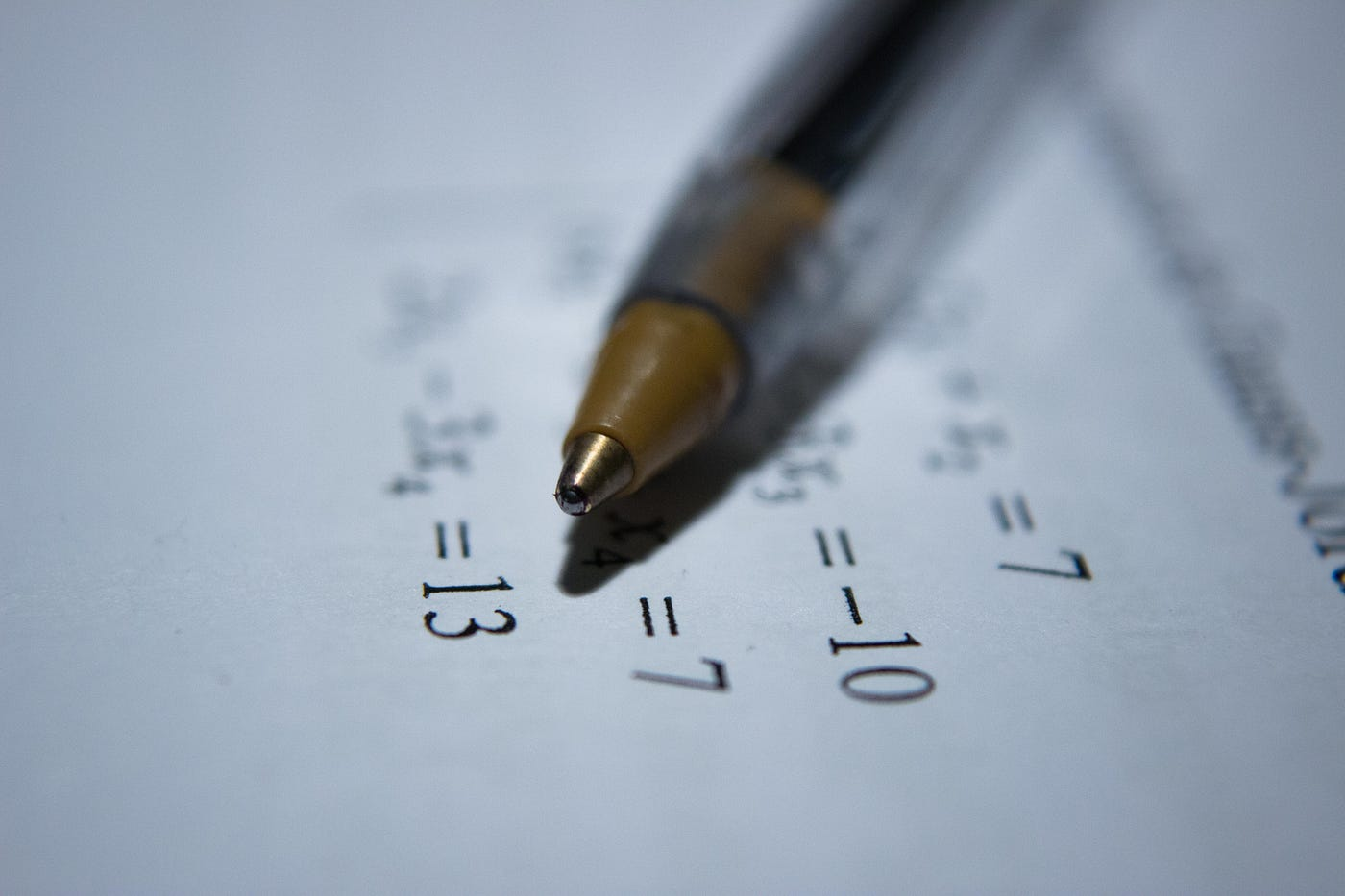# Part 1: Matrix Definitions

## How to better solve systems of equations

As break has just recently finished, I’ve finally been able to start my math project in linear algebra. For now, I’ve simply been reading online and watching YouTube videos on the preliminary information; I have concentrated my work on matrices, particularly how they pertain to solving complex and tedious systems of linear equations.Photo by Antoine Dautry on Unsplash

In this first part of my series documenting my journey, I’ll give brief definitions of some key terms relating to matrices and reflect on what I’ve learned so far.

## What is a Matrix?

A matrix is simply a rectangular array of numbers, typically represented using the letters m and n (m x n) to indicate the number of rows and columns respectively; it can essentially be thought of as a layout of numbers.

In the example above, the matrix A would be 2 rows by 3 columns. Let’s move straight into the part where matrices can help in solving systems of linear equations. I was introduced to solving basic systems of equations (2 equations) a long time ago, and I was typically told to either try substituting or manipulating one of the equations so that the elimination of a variable would be possible. But that only really was possible when the numbers worked well, and when new equations and variables entered the scene, I was lost in tedious work. I had some prior knowledge that hinted at matrices being useful in tackling the more monotonous linear equations questions, but I never really knew how it worked.

## Augmented Matrix

With systems of linear equations, we can represent the coefficients on the variables as well as the constant in each equation as numbers in a matrix. The augmented matrix is extremely useful in the solution process, and it is the starting point to be able to solve systems of linear equations using matrices. However, before we hop into its definition, it is important to understand what the coefficient matrix and the vector of constants are.

• Coefficient Matrix: A coefficient matrix is exactly what it sounds like: it is a matrix containing all of the coefficients on the variables in a system of linear equations. These numbers are laid out in the matrix in the “exact same spot visually” as they appear in the linear equations. Refer to the example below to better see what I mean by this. Also note that the coefficient matrix excludes numbers on the right-hand side of the equals sign.

Here is a sample system of linear equations. It contains 3 variables and 3 equations, so it is certainly solvable by brute force, but we can do better. After taking the coefficients, we obtain the following coefficient matrix.

• Vector of Constants: Simply put, the vector of constants is a matrix that includes the right-hand side of the equations in a system of linear equations. Each equation usually has operations on different “coefficients” of different variables that are equal to a constant. The vector of constants contains those constants. If we use the system of linear equations from the previous example, its vectors of constants would look like this.

The augmented matrix appends these two matrices from left to right, starting with the coefficient matrix.

## Reduced Row-Echelon Form (RREF)

The reduced row-echelon form matrix of a system of linear equations is the key to our solution. It is a special type of matrix that is obtained from performing different row operations on an augmented matrix. We can use the simpler RREF matrix to obtain a new system of linear equations with the same answers as our original system, which is much easier to work with. Here are some characteristics of an RREF matrix.

• If there exists a row where every number is 0, then the row must be under any row with an number that is not 0.
• the leftmost nonzero number is 1.
• the leftmost nonzero number in a row is the only nonzero number in its respective column.

Choosing the correct row operations on the augmented matrix to achieve our RREF matrix is something that I am currently grappling with, and truthfully, it is most practically calculated with a computer. Some examples with 2 by 2 matrices make sense to me, but as the number of columns and rows increase, I find that I have trouble. When I do figure this out, I will make sure to post an update. However, we do have our RREF matrix, our work is almost done. Using this new matrix, we can think of the numbers as the coefficients on our variables of a new system of linear equations that will give us the answers we are looking for (because we used row operations).

This is a very nice and special example because the coefficients on 2 out of the 3 variables in each equation are 0, so we effectively already have our answers.

x1 = -3

x2 = 5

x3 = 2

## Reflections

I must say, learning a whole new topic in math is extremely difficult! I have mainly been teaching myself through articles and YouTube videos; it has been a fun experience so far. At times, I felt slightly lost just because I’ve never been exposed to such a topic in higher-level mathematics. But, I am motivated to learn more, particularly because of the fact that linear algebra has some ties with machine learning, an area of computer science that I am interested in exploring deeper. I hope you found this article useful, and I will be updating on my progress soon!

--

--